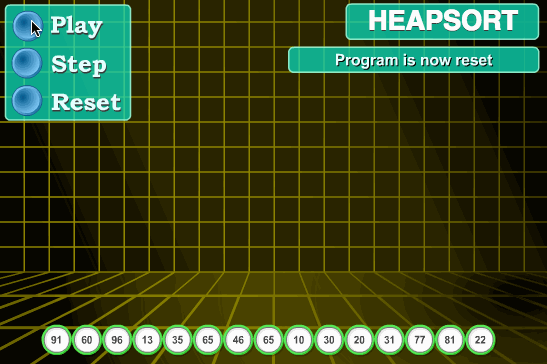# Sorting

Sorting Algorithms with Animations

Sorting Algorithms (Selection Sort, Bubble Sort, Merge Sort, and Quicksort)

Selection Sort, Bubble Sort, and Insertion Sort - Algorithms for Coding Interviews in Python

Sorting is any process of arranging items systematically. In computer science, sorting algorithms put elements of a list in a certain order.### Examples

• Dutch National Flag Problem
• Sort big file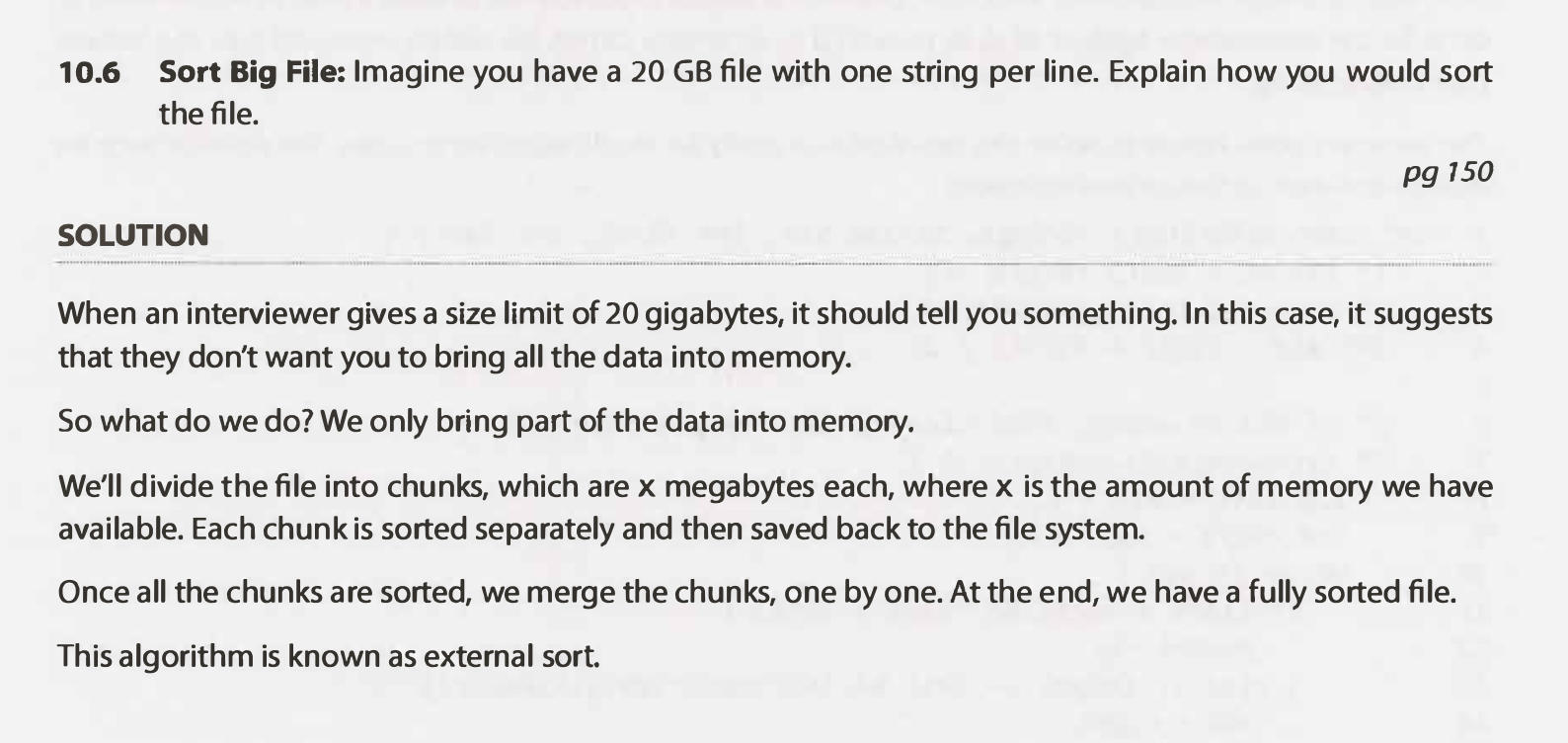• Peaks and Valleys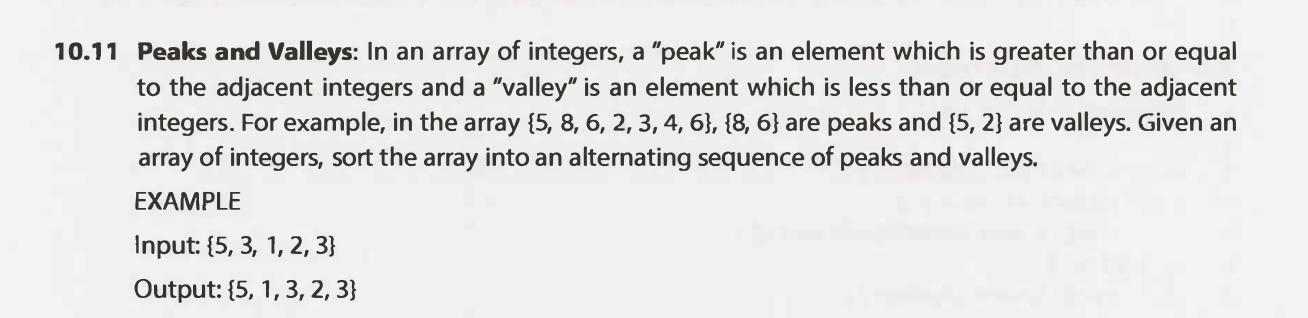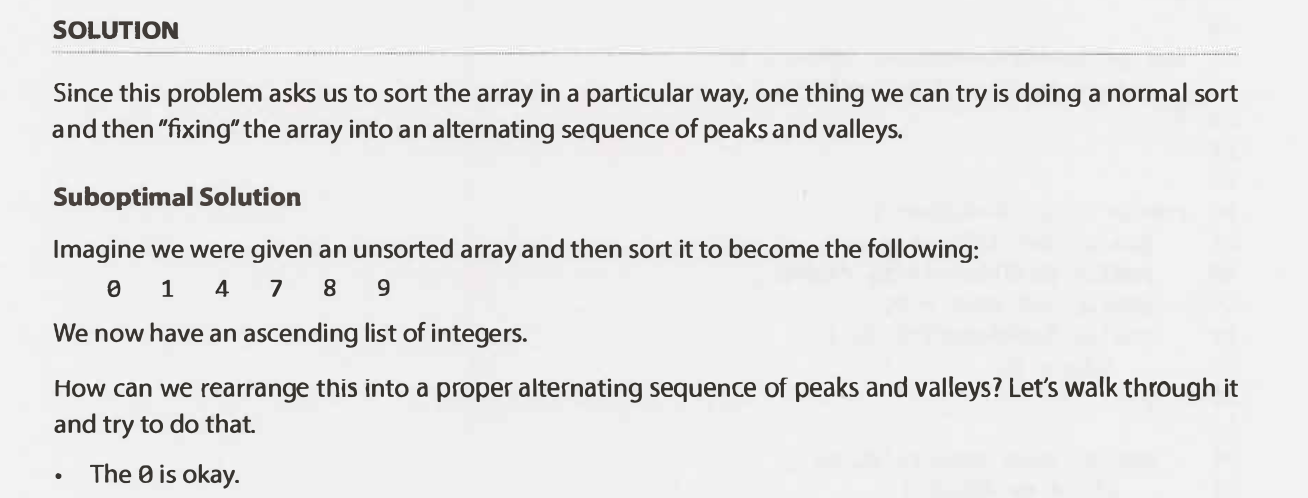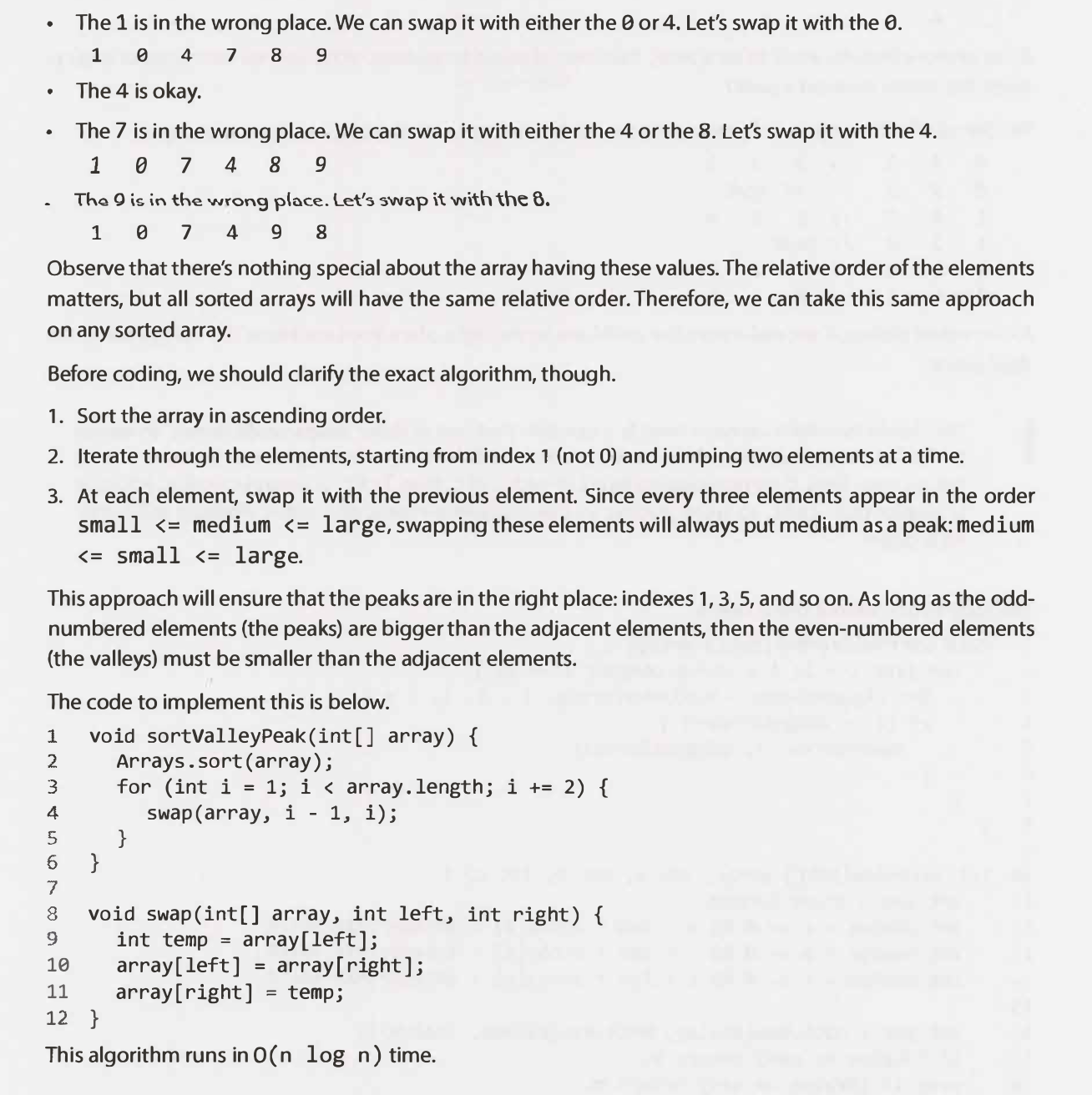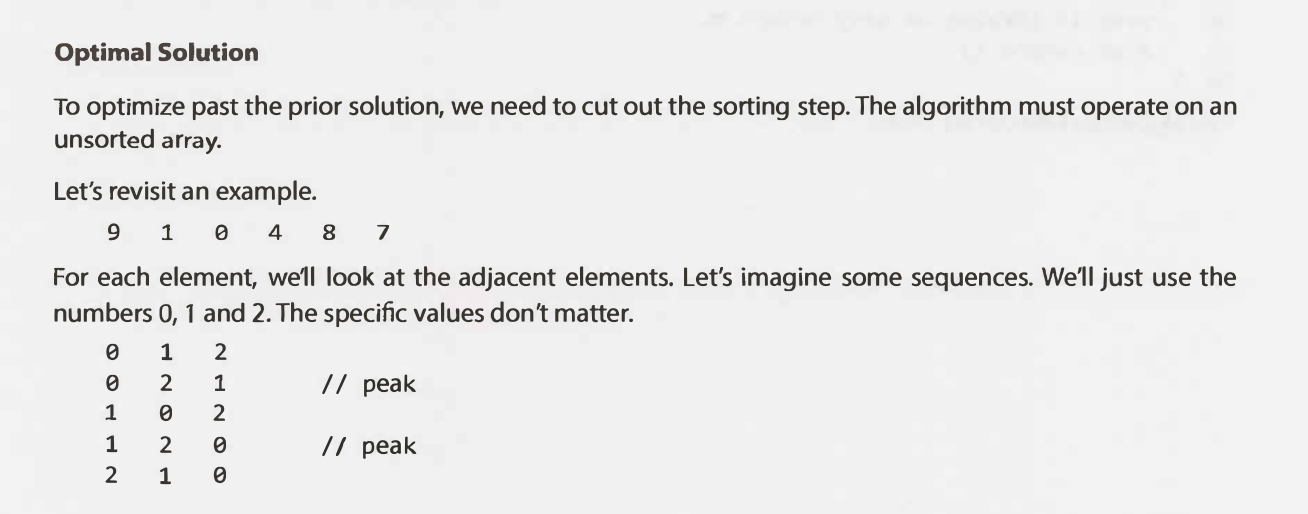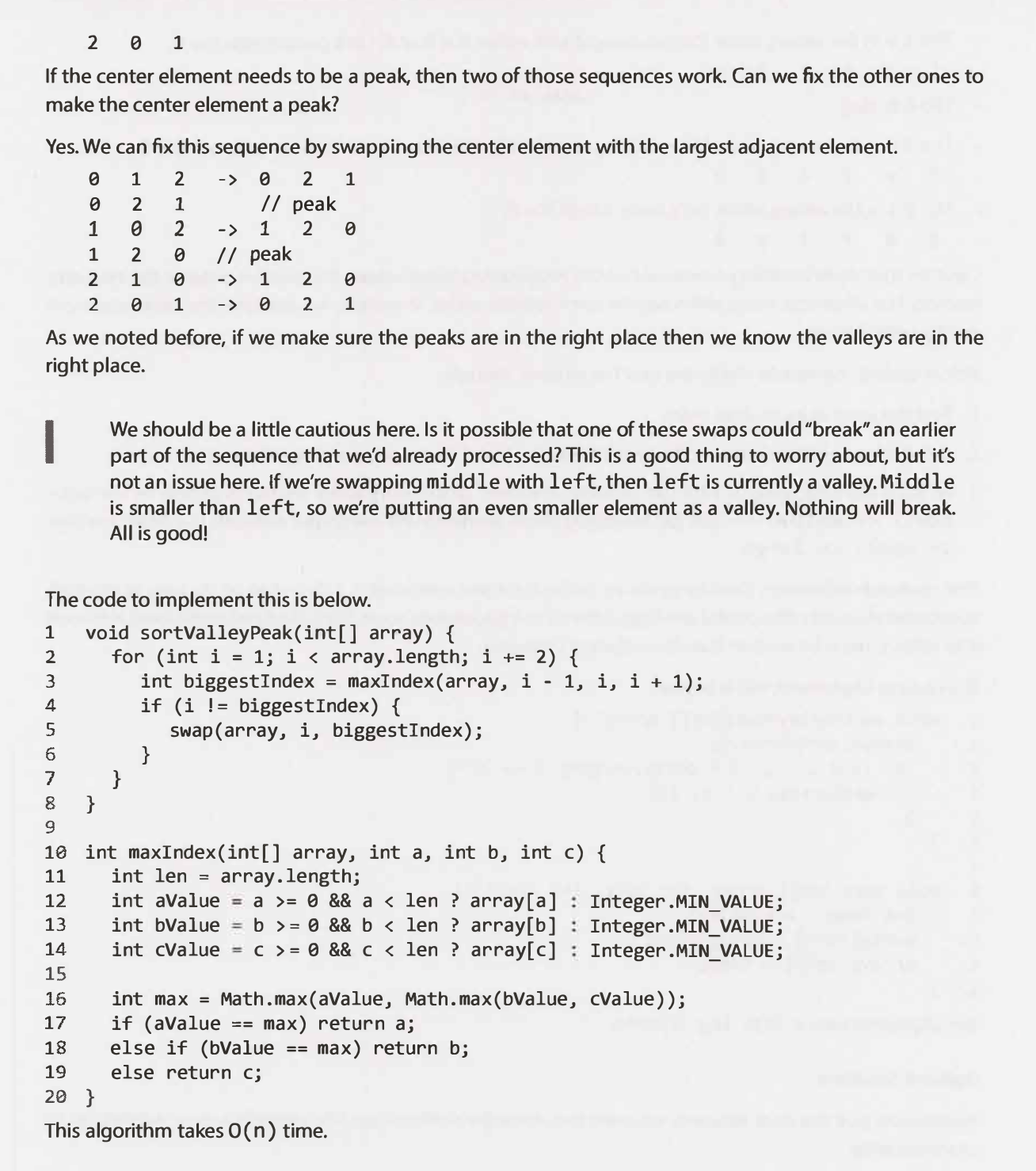• Queue Reconstruction by Height *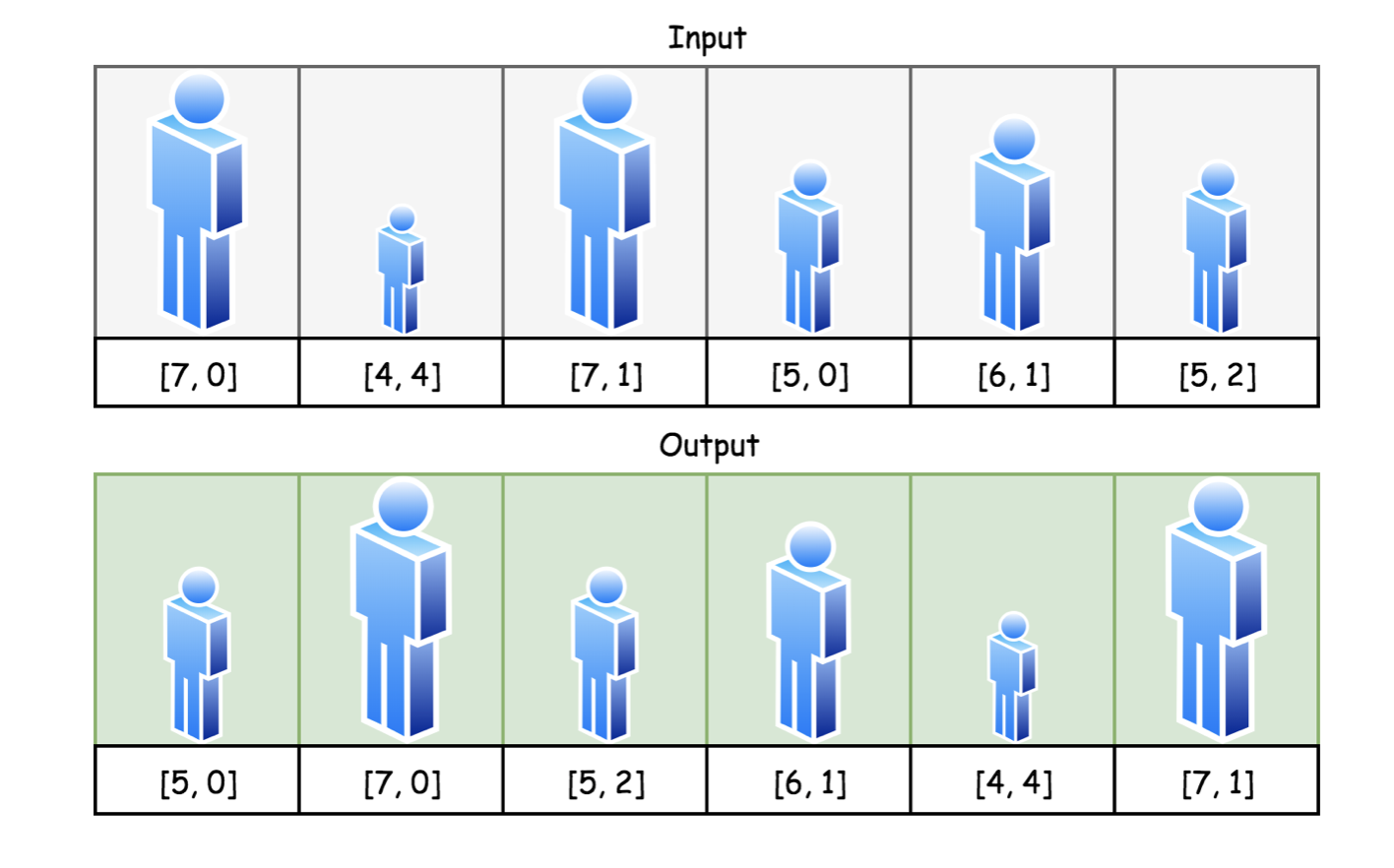"""
406. Queue Reconstruction by Height

You are given an array of people, people, which are the attributes of some people in a queue (not necessarily in order).
Each people[i] = [hi, ki] represents the ith person of height hi with exactly ki other people in front who have a height greater than or equal to hi.
Reconstruct and return the queue that is represented by the input array people.
The returned queue should be formatted as an array queue, where queue[j] = [hj, kj] is the attributes of the jth person in the queue (queue is the person at the front of the queue).

Example 1:
Input: people = [[7,0],[4,4],[7,1],[5,0],[6,1],[5,2]]
Output: [[5,0],[7,0],[5,2],[6,1],[4,4],[7,1]]
Explanation:
Person 0 has height 5 with no other people taller or the same height in front.
Person 1 has height 7 with no other people taller or the same height in front.
Person 2 has height 5 with two persons taller or the same height in front, which is person 0 and 1.
Person 3 has height 6 with one person taller or the same height in front, which is person 1.
Person 4 has height 4 with four people taller or the same height in front, which are people 0, 1, 2, and 3.
Person 5 has height 7 with one person taller or the same height in front, which is person 1.
Hence [[5,0],[7,0],[5,2],[6,1],[4,4],[7,1]] is the reconstructed queue.
Example 2:
Input: people = [[6,0],[5,0],[4,0],[3,2],[2,2],[1,4]]
Output: [[4,0],[5,0],[2,2],[3,2],[1,4],[6,0]]

Constraints:
1 <= people.length <= 2000
0 <= hi <= 106
0 <= ki < people.length
It is guaranteed that the queue can be reconstructed.

https://leetcode.com/problems/queue-reconstruction-by-height
"""

from typing import List
"""
https://www.notion.so/paulonteri/Sorting-c597de5051f1415793ddcf72086aa93d#228cc55cb27b4f08a9d7ffdbb64a2d32
[[1, 4], [2, 2], [3, 2], [4, 0], [5, 0], [6, 0]]
[[4, 0], [5, 0], [2, 2], [3, 2], [1, 4], [6, 0]]

[[1, 4], [2, 2], [3, 2], [4, 0], [5, 0], [6, 0]]
[0,       1,      2,      3,      4,      5]
[1,4] skip 4 free positions
[2, 2], skip 2 free positions
[3, 2], skip 2 free positions
[4, 0], skip 0 free positions
[5, 0], skip 0 free positions
[6, 0], skip 0 free positions

Similar solutions:
- https://leetcode.com/problems/queue-reconstruction-by-height/discuss/89407/Python-Documented-solution-in-O(n*n)-time-that-is-easy-to-understand
- https://leetcode.com/problems/queue-reconstruction-by-height/discuss/167308/Python-solution

"""

class Solution:

def reconstructQueue(self, people: List[List[int]]):
"""
Sort by height then,
push the shorter people to be after taller/same height ones depending on their position
"""
# edge cases
if not people:
return people

# -pos to ensure that people with the same hight but different positions are dealt with
# example; dealing with such -> [[7,0],[7,1]]
sorted_people = [[height, -pos] for height, pos in people]
sorted_people.sort()

queue = [None]*len(people)
for height, neg_pos in sorted_people:
self.place_in_queue(queue, height, neg_pos)

return queue

def place_in_queue(self, queue, height, neg_pos):
"""
Place person in the first valid position
- position must not be occupied
- position is got by skipping -neg_pos times
"""
skips = -neg_pos
for idx, item in enumerate(queue):
if item is not None:
continue
if skips == 0:
queue[idx] = [height, -neg_pos]
return

skips -= 1

• Rank from stream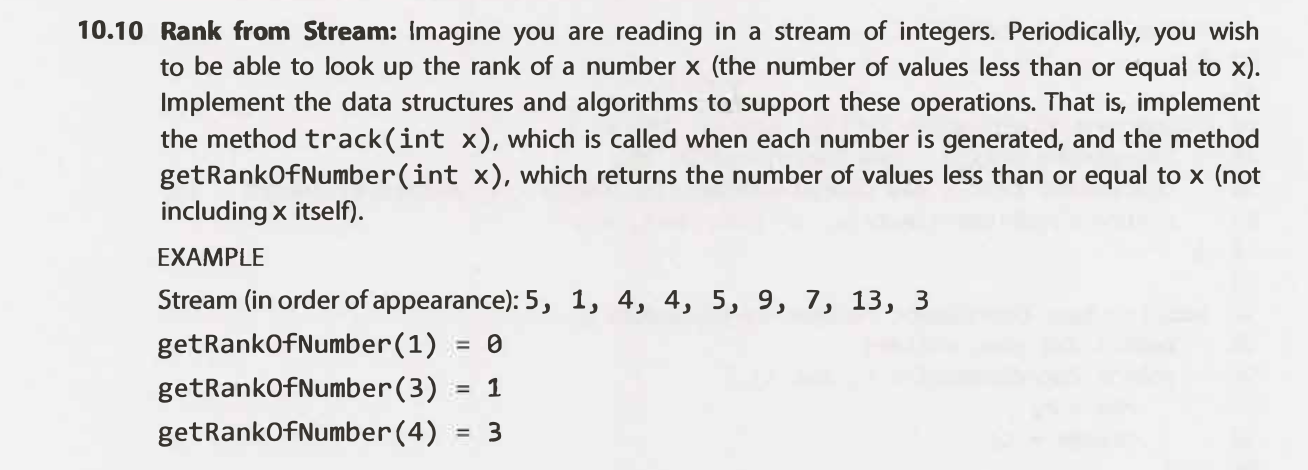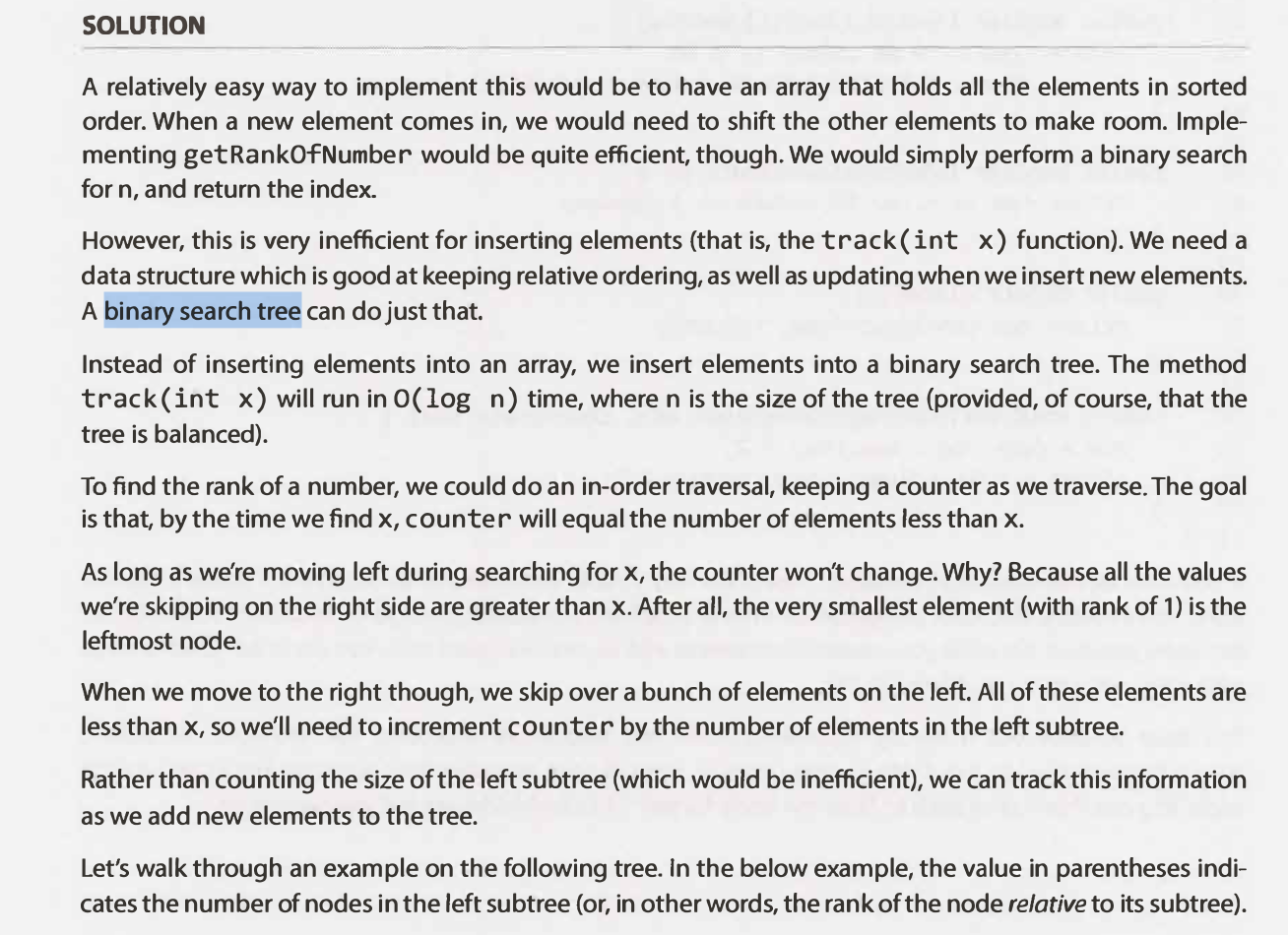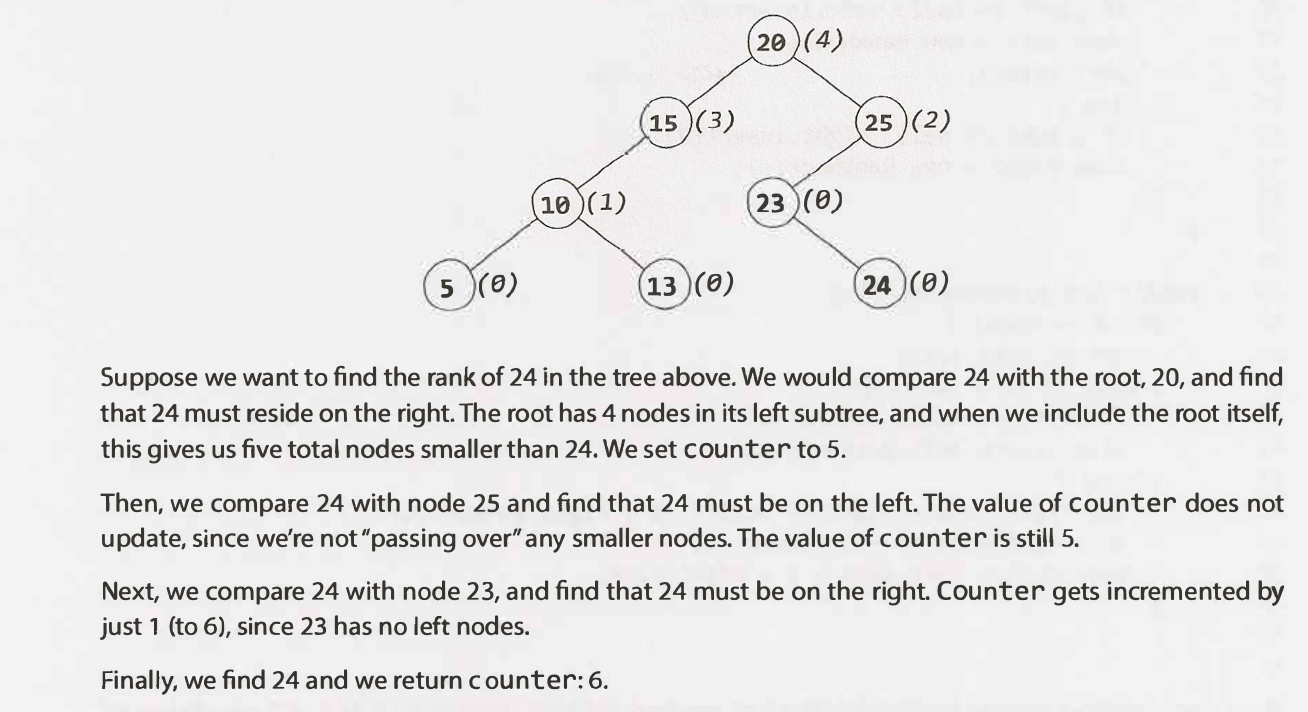## Common operations

### sort() parameters (key & reverse)

The syntax of the sort() method is:

list.sort(key=..., reverse=...)


key

list.sort(key=len)
sorted(list, key=len)

# take second element for sort
def takeSecond(elem):
return elem

# random list
random = [(2, 2), (3, 4), (4, 1), (1, 3)]

# sort list with key
random.sort(key=takeSecond)

# print list
print('Sorted list:', random)
# Sorted list: [(4, 1), (2, 2), (1, 3), (3, 4)]


Examples:

• Merge Intervals

# Bubble Sort

Bubble Sort Tutorials & Notes | Algorithms | HackerEarth

Bubble sort in 2 minutes

Traverse the input array, swapping any two numbers that are out of order and keeping track if you make any swap. Once you arrive at the end of the array, check if you have made any swaps; if not, the array is sorted and you are done; otherwise, repeat the steps laid out in this hint until the array is sorted.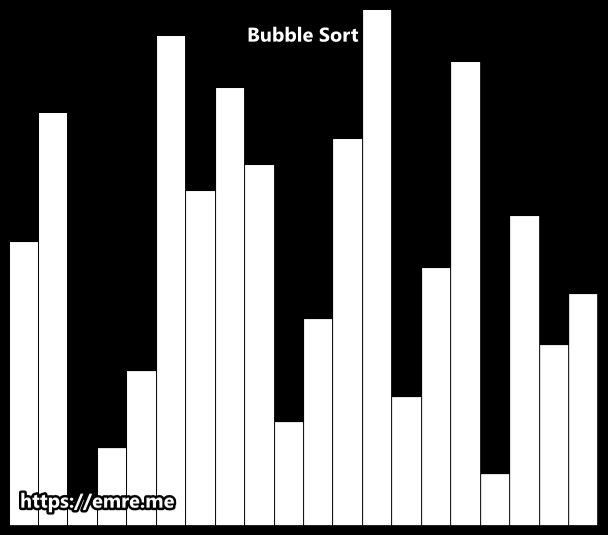# Best: O(n) time | O(1) space -> if already sorted
# Average: O(n^2) time | O(1) space
# Worst: O(n^2) time | O(1) space
def bubbleSort(array):
if len(array) < 2:
return array

is_sorted = False
while not is_sorted:
is_sorted = True

for i in range(len(array)-1):
if array[i] > array[i+1]:
is_sorted = False
# swap
array[i], array[i+1] = array[i+1], array[i]

return array


# Insertion Sort

### Examples

Layman explanation

Have to subarrays (in place):

1. Take an element from the second and insert it to the first.
2. Sort the first using something similar to Bubble sort.

Note: seeing the code makes it way easier to understand it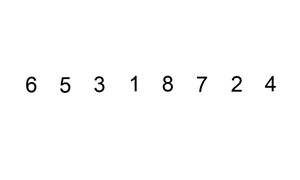Insertion Sort in Action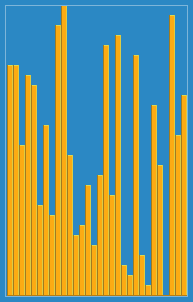Divide the input array into two subarrays in place. - The first subarray should be sorted at all times and should start with a length of 1, while the second subarray should be unsorted. Iterate through the unsorted subarray, inserting all of its elements into the sorted subarray - in the correct position by swapping them into place. Eventually, the entire array will be sorted. Has like a slow bubble sort (for the sorted array).

Explanation for the code: Start with a 'sorted array' of size one at index 0. Insert the element to the right of the sorted array to the sorted array and swap it with other elements till it reaches a balanced position repeat the steps above till you reach the end of the array

# Best: O(n) time | O(1) space -> if already sorted
# Average: O(n^2) time | O(1) space
# Worst: O(n^2) time | O(1) space
def insertionSort(array):

for i in range(1, len(array)):

# # insert a new element(array[i]) to the sorted array
# the sorted array will now end at i
# swap

return array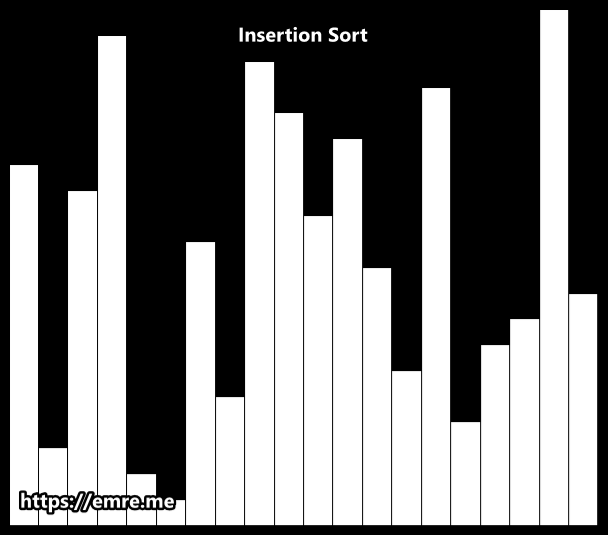# Selection Sort

Divide the input array into two subarrays in place. The first subarray should be sorted at all times and should start with a length of 0, while the second subarray should be unsorted. Select the smallest (or largest) element in the unsorted subarray and insert it into the sorted subarray with a swap. Repeat this process of finding the smallest (or largest) element in the unsorted subarray and inserting it in its correct position in the sorted subarray with a swap until the entire array is sorted.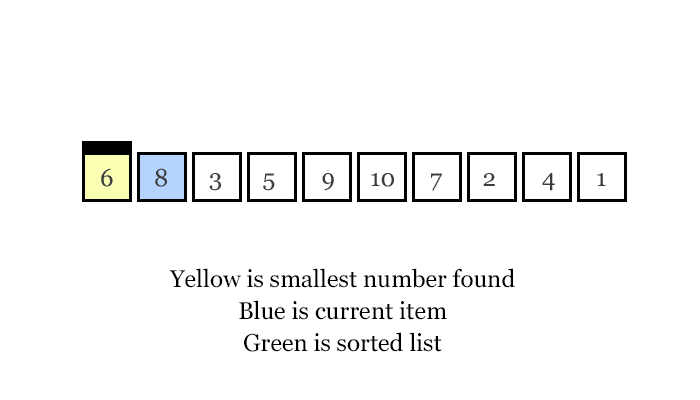# Best: O(n^2) time | O(1) space
# Average: O(n^2) time | O(1) space
# Worst: O(n^2) time | O(1) space
def selectionSort(array):

# select the smallest element and place it at i
for i in range(len(array)-1):

smallest = i
for idx in range(i+1, len(array)):
if array[smallest] > array[idx]:
smallest = idx

# swap
array[i], array[smallest] = array[smallest], array[i]

return array


# Merge Sort?

Merge sort is a recursive divide & conquer algorithm that essentially divides a given list into two halves, sorts those halves, and merges them in order. The base case is to merge two lists of size 1 so, eventually, single elements are merged in order; the merge part is where most of the heavy lifting happens.

The time complexity of merge sort is nlog(n).A recursive merge sort algorithm used to sort an array of 7 integer values.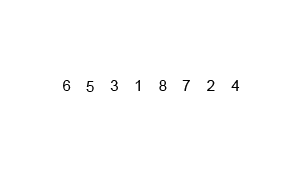# Quick sort

AlgoExpert | Ace the Coding Interviews

Quick sort is the fastest-known, comparison-based sorting algorithm for lists in the average case.It is an algorithm of Divide & Conquer type.

It has 3 steps:

1. We first select an element which we will call the pivot from the array.
2. Move all elements that are smaller than the pivot to the left of the pivot; move all elements that are larger than the pivot to the right of the pivot. This is called the partition operation.
3. Recursively apply the above 2 steps separately to each of the sub-arrays of elements with smaller and bigger values than the last pivot.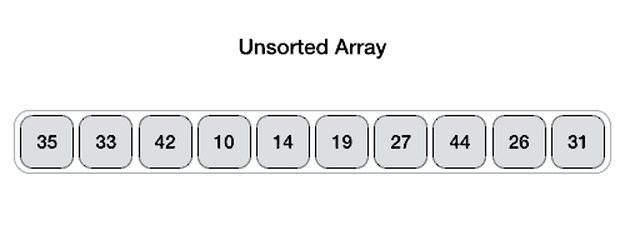Quick Sort works by picking a "pivot" number from an array, positioning every other number in the array in sorted order with respect to the pivot (all smaller numbers to the pivot's left; all bigger numbers to the pivot's right), and then repeating the same two steps on both sides of the pivot until the entire array is sorted.

Pick a random number from the input array (the first number, for instance) and let that number be the pivot. Iterate through the rest of the array using two pointers, one starting at the left extremity of the array and progressively moving to the right, and the other one starting at the right extremity of the array and progressively moving to the left. As you iterate through the array, compare the left and right pointer numbers to the pivot. If the left number is greater than the pivot and the right number is less than the pivot, swap them; this will effectively sort these numbers with respect to the pivot at the end of the iteration. If the left number is ever less than or equal to the pivot, increment the left pointer; similarly, if the right number is ever greater than or equal to the pivot, decrement the right pointer. Do this until the pointers pass each other, at which point swapping the pivot with the right number should position the pivot in its final, sorted position, where every number to its left is smaller and every number to its right is greater.

Repeat the process mentioned on the respective subarrays located to the left and right of your pivot, and keep on repeating the process thereafter until the input array is fully sorted.

"""
Quick Sort:

Quick Sort works by picking a "pivot" number from an array, positioning every other number in the array in sorted order with respect to the pivot (all smaller numbers to the pivot's left;
all bigger numbers to the pivot's right), and then repeating the same two steps on both sides of the pivot until the entire array is sorted.

Pick a random number from the input array (the first number, for instance) and let that number be the pivot.
Iterate through the rest of the array using two pointers, one starting at the left extremity of the array and progressively moving to the right,
and the other one starting at the right extremity of the array and progressively moving to the left.
As you iterate through the array, compare the left and right pointer numbers to the pivot.
If the left number is greater than the pivot and the right number is less than the pivot, swap them; this will effectively sort these numbers with respect to the pivot at the end of the iteration.
If the left number is ever less than or equal to the pivot, increment the left pointer;
similarly, if the right number is ever greater than or equal to the pivot, decrement the right pointer.
Do this until the pointers pass each other, at which point swapping the pivot with the right number should position the pivot in its final,
sorted position, where every number to its left is smaller and every number to its right is greater.

Repeat the process mentioned on the respective subarrays located to the left and right of your pivot, and keep on repeating the process thereafter until the input array is fully sorted.
"""

# Average: O(nlog(n)) time | O(log(n)) space
# Worst: O(n^2) time | O(log(n)) space
def quickSort(array):
quick_sort_helper(array, 0, len(array)-1)
return array

def quick_sort_helper(array, start, end):
if start >= end:
return

pivot = start
# # position every number in the array in sorted order with respect to the array[pivot]
#   left and right to stop at a place where: left >= pivot & right <= pivot
left = start + 1
right = end
while left <= right:
# # check if can swap
if array[left] > array[pivot] and array[right] < array[pivot]:
array[left], array[right] = array[right], array[left]

# # cannot swap
elif array[left] <= array[pivot]:  # wait to be swapped
left += 1
else:  # if array[right] >= array[pivot]:  # wait to be swapped
right -= 1

# # place pivot at correct position
# we know that once the sorting is done, the number at left >= pivot & right <= pivot
#   smaller values go to the left of array[pivot]
array[pivot], array[right] = array[right], array[pivot]

# # sort to the left & right of array[pivot]
if (right-1 - start) < (end - right+1):
quick_sort_helper(array, start, right-1)
quick_sort_helper(array, right+1, end)
else:
quick_sort_helper(array, right+1, end)
quick_sort_helper(array, start, right-1)


Quick Select

# Heap Sort?

Heap sort in 4 minutes

Sorting Algorithms with Animations

Heap sort is the improved version of the Selection sort, which takes advantage of a heap data structure rather than a linear-time search to find the max value item. Using the heap, finding the next largest element takes O(log(n)) time, instead of O(n) for a linear scan as in simple selection sort. This allows heap sort to run in O(n log(n)) time, and this is also the worst case complexity.